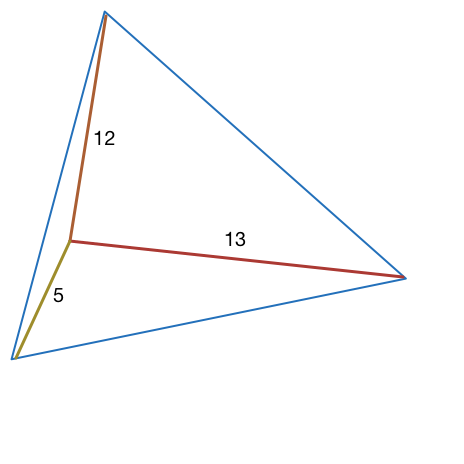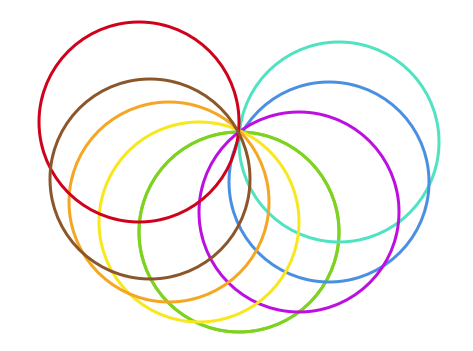Geometry

# Geometry Warmups: Level 4 ChallengesLet $ABC$ an equilateral triangle, $P$ a point inside the triangle such as $AP=5, BP=12$ and $CP=13$. Find the area of $\triangle ABC$.

Evaluate

$\frac{241}{2-\cot^{2} (9^{\circ})}+\frac{241}{2-\cot^{2} (27^{\circ})}+\frac{241}{2-\cot^{2} (45^{\circ})}\\ +\frac{241}{2-\cot^{2} (63^{\circ})}+\frac{241}{2-\cot^{2} (81^{\circ})} .$

$(x_1 - x_2)^2 + \left ( \sqrt{2-x_1 ^2} - \frac {9}{x_2} \right )^2$

What is the minimum value of the above expression where $x_1 \in (0, \sqrt{2})$ and $x_2 \in R^+$A person is bored waiting in line. He draws 1000 congruent circles in the plane, all passing through a fixed point, P. What is the largest number of regions into which these circles can split the plane? (Include the region outside the circles in your count)

###### adapted from the Mandlebrot competition

In the interval $[ 0, 2\pi ]$, how many solutions are there to

$\cos^2 x + \cos^2 2x + \cos^2 3x = 1?$

×